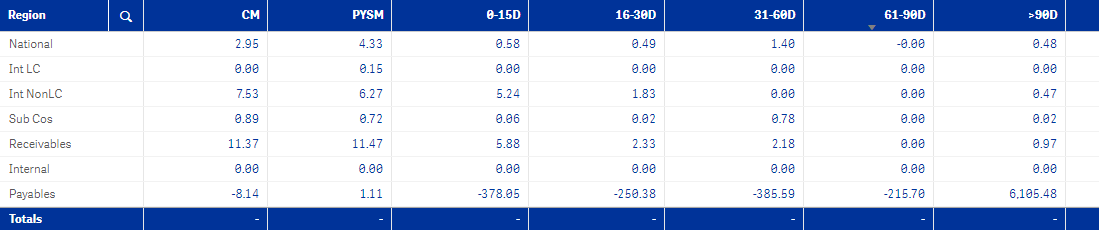# New to Qlik Sense

If you’re new to Qlik Sense, start with this Discussion Board and get up-to-speed quickly.

Announcements
Support Cases coming to Qlik Community Oct. 4! Start chats, open cases, explore resources: READ DETAILS
cancel
Showing results for
Did you mean:Contributor II

## If condition in set expression

Hie...I want if condition to be applied in the set expression can anyone please help me out.

Set expression:

if(NO=7,(SUM(Aggr(Sum({<[P.HOLD_FLAG]={'N'},[P.DUE_DATE]={'<=\$(vMaxDate)'},[P.Division]=Division>}[P.PAYABLE_AMOUNT]),

[P.DUE_DATE],Region)))/vDenom

If condition to be included in set:

(vMaxDate-[P.DUE_DATE])>=0 and (vMaxDate-[P.DUE_DATE])<=15

4 RepliesMVP

Not sure I understand... would you be able to provide a sample?Contributor II
Author

Hie sunny,

Following is the example:

payable:

hold_flag,

amount,

status,

due_date,

p.division,

region

Island_table:

date,

division

created a varialbe: vMaxDate=max(date)

for selcetion fields on the sheet I am using Island table fiels, So I am using the fields in set to compare the payable field.

the following is the expression:

(SUM(Aggr(Sum({<[hold_flag]={'N'},[p.division]=division>}amount),due_date,region)))

now i want I more condition that the amount following in 0-15, 16-30,31-60,61-90,>90 days with respect to the selection made on the sheet.

(vMaxDate-due_date)>=0 and (vMaxDate-due_date)<=15

Following is the screenshot:sum of days bucket should be CM.MVP

So what exactly is the issue you are running into?Contributor III

Good Morning,

If I understood, it appears an "Intersection" is needed in the set.  Below is my example as I just worked on this yesterday.

Hope it helps.

What this is doing is a "intersection".  It states (count (IF the Intersection is >=1 and <=72). (THE * is the intersection of "both SETS".

Count({\$<HoursBetweenEDVisits={">=1"}>
*
<
HoursBetweenEDVisits={"<=72"}>
}
CSN)Tags
Community Browser